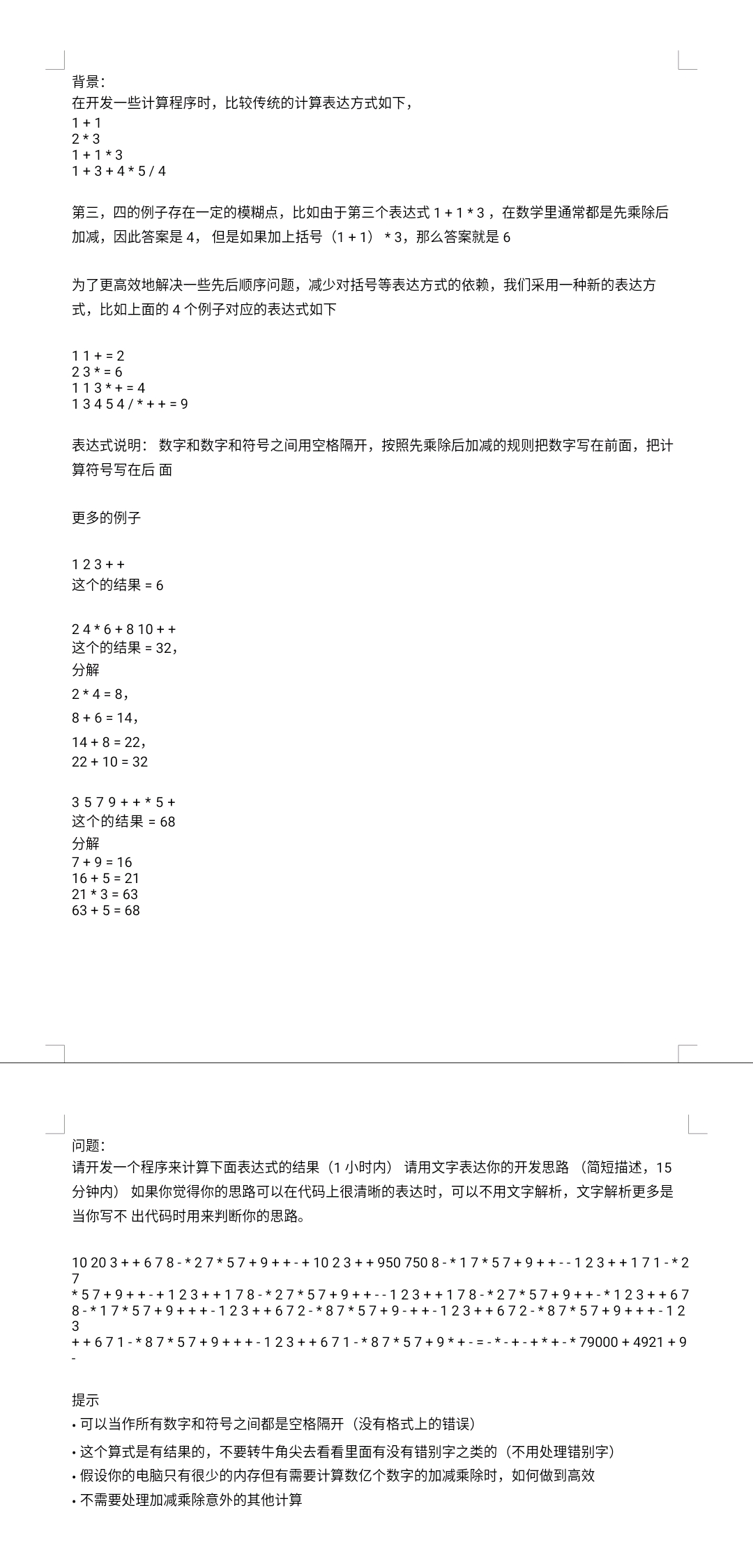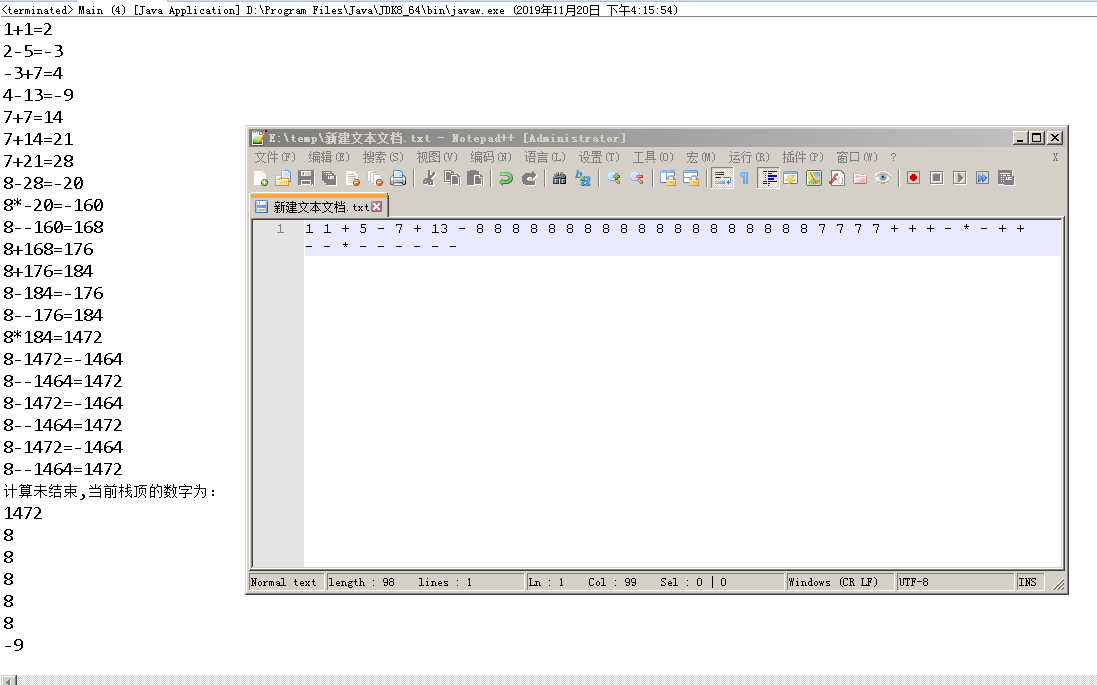•闪存
•博客
• 发言小组
• 投递新闻
• 提问博问
• 添加收藏
•文库

# 数学问题 编程题

0悬赏园豆：100 [已解决问题] 解决于 2019-11-23 08:231+1231+131+3+45/4第三，四的例子存在一定的模糊点，比如由于第三个表达式1+13，在数学里通常都是先乘除后加减，因此答案是4，但是如果加上括号（1+1）3，那么答案就是6为了更高效地解决一些先后顺序问题，减少对括号等表达方式的依赖，我们采用一种新的表达方式，比如上面的4个例子对应的表达式如下
11+=223
=6113+=413454/++=9表达式说明：数字和数字和符号之间用空格隔开，按照先乘除后加减的规则把数字写在前面，把计算符号写在后面

123++

2
4=8，
8+6=14，
14+8=22，22+10=323579++5+

7+9=1616+5=2121
3=6363+5=68

10203++678-2757+9++-+1023++9507508-1757+9++--123++171-2
57+9++-+123++178-2757+9++--123++178-2757+9++-123++678-1757+9+++-123++672-8757+9-++-123++672-8757+9+++-12
++671-
8757+9+++-123++671-8757+9+-=--+-++-*79000+4921+9

.可以当作所有数字和符号之间都是空格隔开（没有格式上的错误）
·这个算式是有结果的，不要转牛角尖去看看里面有没有错别字之类的（不用处理错别字）
·假设你的电脑只有很少的内存但有需要计算数亿个数字的加减乘除时，如何做到高效
.不需要处理加减乘除意外的其他计算0

C语言

``````#include <stdio.h>
#include <string.h>
double cal(const char *s)
{
double stack;
char s2;
int stack_top = -1;
int n;

while(sscanf(s, "%19s%n", s2, &n) == 1) {
if(strcmp(s2, "+") == 0) {
stack[stack_top-1] += stack[stack_top];
stack_top--;
} else if(strcmp(s2, "-") == 0) {
stack[stack_top-1] -= stack[stack_top];
stack_top--;
} else if(strcmp(s2, "*") == 0) {
stack[stack_top-1] *= stack[stack_top];
stack_top--;
} else if(strcmp(s2, "/") == 0) {
stack[stack_top-1] /= stack[stack_top];
stack_top--;
} else {
sscanf(s2, "%lf", &stack[++stack_top]);
}
s += n;
}
return stack[stack_top];
}

int main(int argc, char **argv)
{
printf(" = %lf\n", cal(argv));
return 0;
}``````

Python

``````#!/usr/bin/python3
from functools import reduce
def cal(s):
return reduce(lambda r,n:r[:-2]+[eval(str(r[-2])+n+str(r[-1]))] if n in ('+','-','*','/') else r + [float(n)],s.split(), [])
print(cal('3 4 5 / -'))``````

0http://115.28.130.106/files/java/%E9%80%86%E6%B3%A2%E5%85%B0%E5%BC%8F/

您需要登录以后才能回答，未注册用户请先注册